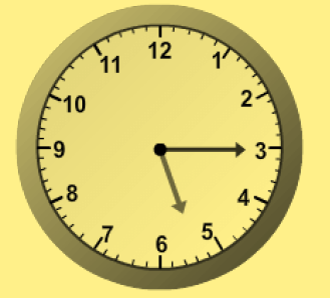1
visibility

What time does the clock below show?• A

5:15

• B

4:15

• C

6:15

• D

5:45

The correct answer is 5:15

Solution:

Consider the clock below:• The hour hand is between 5 and 6, so it is 5 hours.
• The minute hand is at 3. So, if we begin at 12 and count up by fives until we reach 3. It is 15 minutes.

So, the time shown by the clock is 5:15